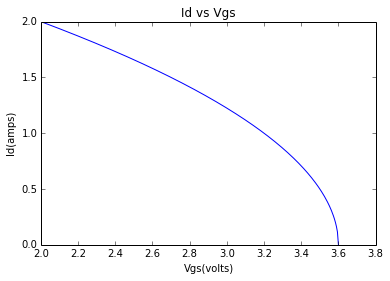# Chapter 5: Discrete Linear Power Amplifier¶

### Example 5.1,Page 215¶

In :
#finding Id vs Vgs

#initialisation of variable
from math import pi,tan,sqrt,sin,cos,acos,atan
%matplotlib inline
import numpy as np
import matplotlib.pyplot as plt
Vth=3.6;
Vgs=4;#voltage
#volt change beyond 3.6 causes a major increase in Id as it is cut off voltage

#result
print('Id=0 from 0 to 2 so not shown in the graph')
x=np.linspace(2,3.6,300);
y=(-2.5*(x-3.6))**.5;
plt.plot(x,y)
plt.xlabel('Vgs(volts)');
plt.ylabel('Id(amps)');
plt.title('Id vs Vgs');

Id=0 from 0 to 2 so not shown in the graph### Example 5.2,Page 217¶

In :
#finding drain current

#initialisation of variable
from math import pi,tan,sqrt,sin,cos,acos,atan
V=4.5;#voltage
T=25;#degreeC
Id=3.8;

#result
print "drain current is",round(Id,2), "A"
print('Vth=4V is assumed')

drain current is 3.8 A
Vth=4V is assumed


### Example 5.3,Page 219¶

In :
#finding drain current of IRF530N

#initialisation of variable
from math import pi,tan,sqrt,sin,cos,acos,atan
Vgs=-5;#voltage
Vthl=-4;
Vthu=-2;
Id=2.3;#current

#result
print('MOSFET is IRF530N')
print "lower limit of Vth is",round(Vthl,2), "V"
print "upper limit of Vth is",round(Vthu,2), "V"
print "current is",round(Id,2), "A"

MOSFET is IRF530N
lower limit of Vth is -4.0 V
upper limit of Vth is -2.0 V
current is 2.3 A


### Example5.5,Page 225¶

In :
#finding Pq,Pl,Ps,resistance,load voltage

#initialisation of variable
from math import pi,tan,sqrt,sin,cos,acos,atan
R1=22.0;#resistance
Vg=3.6;#voltage
Vd=56.0;
G=.98;#gain
Vi=40.0;
Vp=36.5;

#calculation
Vr=Vd-Vg;
Ir=Vr/R1;
R2=Vg/Ir;
Va=(R1/(R1+R2))*Vi;
Vl=G*Va;
Il=Vp/Rl;
Pl=Vp*4.6/4;
Ps=Vd*4.6/pi;
Pq=Ps-Pl;

#result
print "resistance is",round(R2,2), "kohm"
print "Pq is",round(Pq,2), "watt"
print "Ps is",round(Ps,2), "watt"
print "Pl is",round(Pl,2), "watt"

resistance is 1.51 kohm
Pq is 40.02 watt
Ps is 82.0 watt
Pl is 41.97 watt


### Example 5.6,Page 232¶

In :
#finding resistance and current

#initialisation of variable
from math import pi,tan,sqrt,sin,cos,acos,atan
R1=22.0;#resistance
V1=56.0;#voltage
V2=2.0;#voltage

#calculation
I=(V1-V2)/R1;
R2=V2/I;

#result
print "current is",round(I,2), "mA"
print "resistance2 is",round(R2*1000,3), "ohm"
print('pick R2=R3=820ohm R1=R4=22 kohm')

current is 2.45 mA
resistance2 is 814.815 ohm
pick R2=R3=820ohm R1=R4=22 kohm


### Example 5.7,Page 234¶

In :
#finding load voltage

#initialisation of variable
from math import pi,tan,sqrt,sin,cos,acos,atan
Vi=350.0;#voltage
f=100.0;#frequency
Rf=10000.0;#resistance
Ri=520.0;

#calculation
Vp=(1+(Rf/Ri))*Vi*2**.5;

#result

load voltage is 10.01 V


### Example 5.8,Page 238¶

In :
#designing amplifier

#initialisation of variable
from math import pi,tan,sqrt,sin,cos,acos,atan
P=50.0;#power
Z=4.7#impedence
R=4.0;#resistance
Ta=40.0;#degreeC
Tj=140.0;#degreeC
Vd=28.0;
R2=22.0;

#calculation
Vr=(P*R)**.5;
Vp=Vr*2**.5;
Av=-Vr/1.23;
Rf=-Av*Z;
I=(Vd-2)/R2;
Vm=.63*Vd;
Ip=Vm/R;
Ps=Vd*Ip/pi;
Pl=Ip**2/2*R;
Pq=round(Ps)-Pl/2;
Qs=(Tj-Ta)/Pq-2.1;

#result
print "load rms voltage is",round(Vp,2), "V"
print "resistance is",round(Rf,2), "kohm"
print "current is",round(I,2), "mA"
print "supply power is",round(Ps,2), "watt"
print "power is",round(Pq,3), "W"
print "thermal resistance is",round(Qs,2), "degreC/W"

load rms voltage is 20.0 V
resistance is 54.04 kohm
current is 1.18 mA
supply power is 39.3 watt
power is 19.552 W
thermal resistance is 3.01 degreC/W


### Example 5.9,Page 243¶

In :
#finding load current,output voltage

#initialisation of variable
from math import pi,tan,sqrt,sin,cos,acos,atan
Vi=7.5e-3;#voltage
Ib=800e-9;#current
R=53.9e3;#resistance

#calculation
Vo=11.5*Vi+Ib*R;
Id=Vo/4;

#result
print "output voltage is",round(Vo*1000,2), "mV"

output voltage is 129.37 mV


### Example 5.10,Page 249¶

In :
#finding resistance,voltage,power

#initialisation of variable
from math import pi,tan,sqrt,sin,cos,acos,atan
G=6.4;#A/V
I=5.0;#current
Pq=9.8;#W
Tj=140.0;
Ta=40.0;
R1=.33;

#calculation
R=2/G;
Im=I/3;
Vr=Im*R1;
P=Vr*Im/4;
Qs=(Tj-Ta)/Pq-2.1;

#result
print "resistance is",round(R,2), "ohm"
print('thus pick a .33ohm rsistance')
print "voltage is",round(Vr,2), "V"
print "power is",round(P,2), "W"
print "thermal resistance is",round(Qs,2), "degreeC/W"

resistance is 0.31 ohm
thus pick a .33ohm rsistance
voltage is 0.55 V
power is 0.23 W
thermal resistance is 8.1 degreeC/W


### Example 5.11,Page 251¶

In :
#finding limit current

#initialisation of variable
from math import pi,tan,sqrt,sin,cos,acos,atan
P=200;#power
R=8;#ohm

#calculation
Il=(P/R)**.5*2**.5;
Ilm=1.2*Il;

#result
print "limit level current is",round(Ilm,2), "A"

limit level current is 8.49 A


### Example 5.12,Page 253¶

In :
#finding resistance,power,temperature

#initialisation of variable
from math import pi,tan,sqrt,sin,cos,acos,atan
I=6;#current
V=.6;#voltage
D=.5;#duty cycle
T=40;#temperature

#calculation
Rs=V/I;
Pr=D*V*I;
Vp=28;
Pm=D*Vp*I;
Tj=T+Pm*5.1;

#result
print "resistance is",round(Rs,2), "ohm"
print "power is",round(Pr,2), "W"
print "MOSFET power is",round(Pm,2), "W"
print "temperature is",round(Tj,2), "degreeC"

resistance is 0.1 ohm
power is 1.8 W
MOSFET power is 84.0 W
temperature is 468.4 degreeC


### Example 5.13,Page 255¶

In :
#finding maximum safe temperature

#initialisation of variable
from math import pi,tan,sqrt,sin,cos,acos,atan
T=130;#temperature
P=19.5;#power

#calculation
Ts=T-P*2.1;

#result
print "maximum safe temperature is",round(Ts,2), "degreeC"

maximum safe temperature is 89.05 degreeC


### Example 5.14,Page 257¶

In :
#finding 3 powers and current across resistance

#initialisation of variable
from math import pi,tan,sqrt,sin,cos,acos,atan
V=15.0;#voltage
f=300.0;#frequency
L=4.7;#inductance
Vdc=28;#V
Pr=12.5;

#calculation
Xl=2*pi*f*L;
I=Vr/8*sqrt(2);
Psupply=Vdc*I/pi;
Pq=Psupply-Pr/2;

#result
print "reactance is",round(Xl/1000,2), "ohm"
print "voltage across resistor is",round(Vr,2), "V"
print "-48 is the angle of the voltage in degrees";
print "power dissipated by load is",round(Pr,2), "watts"
print "current across the resistance is",round(I,2), "A"
print "power supply is",round(Psupply,2), "W"
print "power dissipated by transistor is",round(Pq,2), "watts"

reactance is 8.86 ohm
voltage across resistor is 10.03 V
-48 is the angle of the voltage in degrees
power dissipated by load is 12.5 watts
current across the resistance is 1.77 A
power supply is 15.8 W
power dissipated by transistor is 9.55 watts